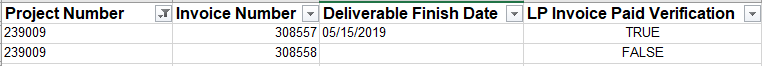# How to tell if Multiple Invoices have all been processed

I've created a sheet that we use to track our projects.

For each project there could be one invoice we need to pay, or multiple, as the project progresses when the invoice is processed and the checks are sent out to our partners there is a column that indicates the date the invoice processing is complete. I've created a verification column as a check box that populates when the completion date is populated.

So we have 4 columns Project Number, Invoice Number, Completion Date, Completion Date Verification.

The goal we're trying to reach is for each project line, if ALL invoices per project have a completion date, return true, else return false.

If we added another verification column to achieve our goal would we need to use an index to accomplish this? A vlookup wouldn't work as we could have anywhere from 1-X amount of invoices per project, would we need to use an array? does that functionality exist in SmartSheets?

Project Number    Invoice Number    Completion Date    Completion Date Verification

239009                308557                  05/15/2019                  True

239009                308558                                                      False• How are you wanting to display the end result?

The basics of this would be comparing two COUNTIFS functions within an IF statement. Something along the lines of

=IF(the count of true's for specific project = the count of lines for specific project, true)

.

COUNTIFS([Project Number Verification]:[Project Number Verification], ######, [Completion Date Verification]:[Completion Date Verification], TRUE)

Will give you how many are true for that project number.

.

Will tell you how many rows contain that project number.

.

If both of those numbers match, then that means they are all TRUE.

=IF(COUNTIFS([Project Number Verification]:[Project Number Verification], ######, [Completion Date Verification]:[Completion Date Verification], TRUE) = COUNTIFS([Project Number Verification]:[Project Number Verification], ######), TRUE)

• Gotcha yeah that's much easier than I what I was thinking I'd have to do, thank you so much for the help, my actual formula is below in case it helps anyone.

=IF(COUNTIFS([Project Number]:[Project Number], [Project Number]1, [LP Invoice Paid Verification]:[LP Invoice Paid Verification], true) = COUNTIFS([Project Number]:[Project Number], [Project Number]1), true, false)

• Happy to help!## Help Article Resources

Want to practice working with formulas directly in Smartsheet?

Check out the Formula Handbook template!